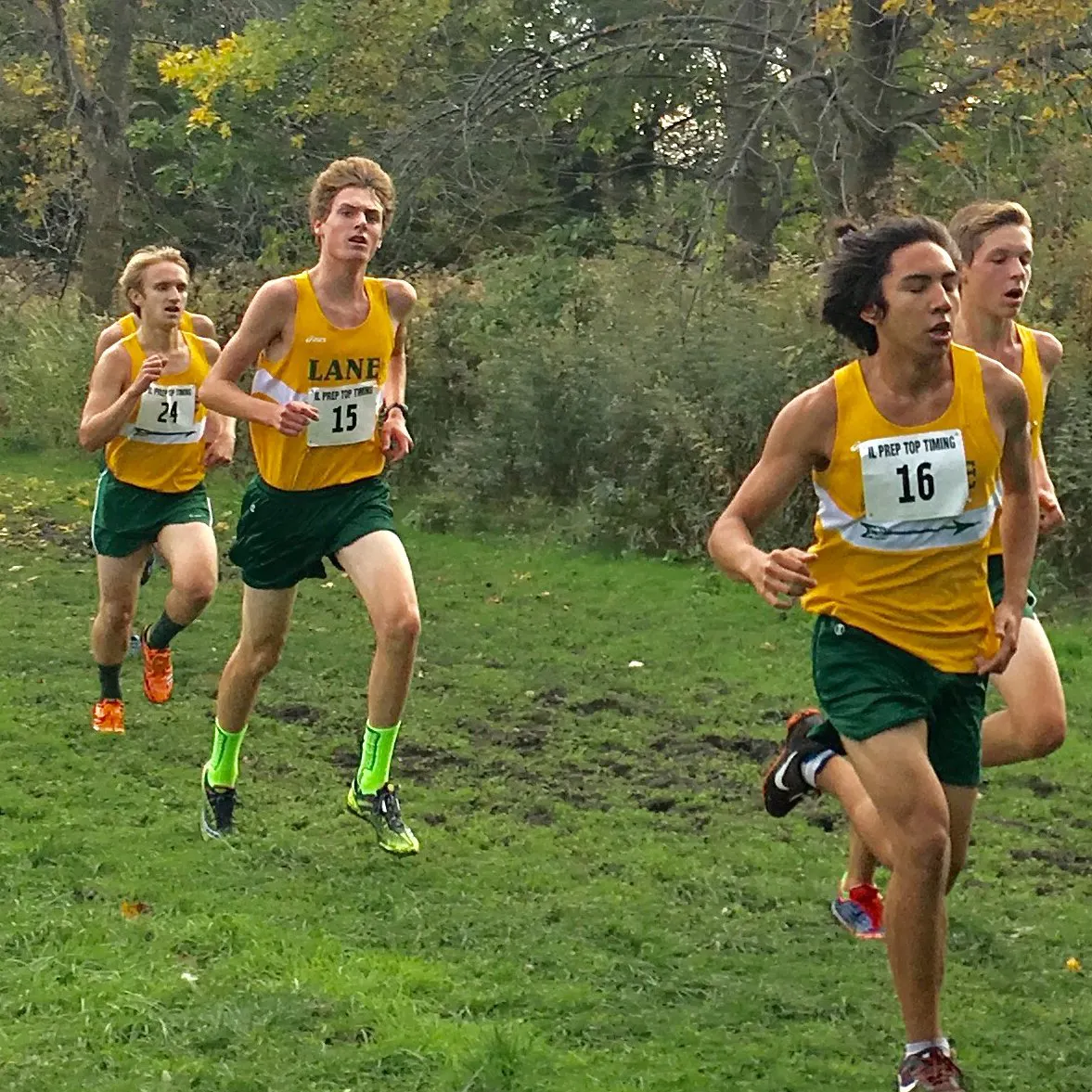Published

# Interactive Kid's Toy

A toy for infants with many interactive features such as buttons, knobs, sound, and motion.

IntermediateOver 1 day540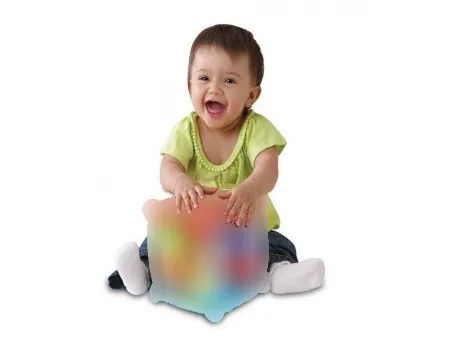## Things used in this project

### Hardware components

 Slide Pot - X-Large (10k Linear Taper)
×1
 Slide Potentiometer Knob
×1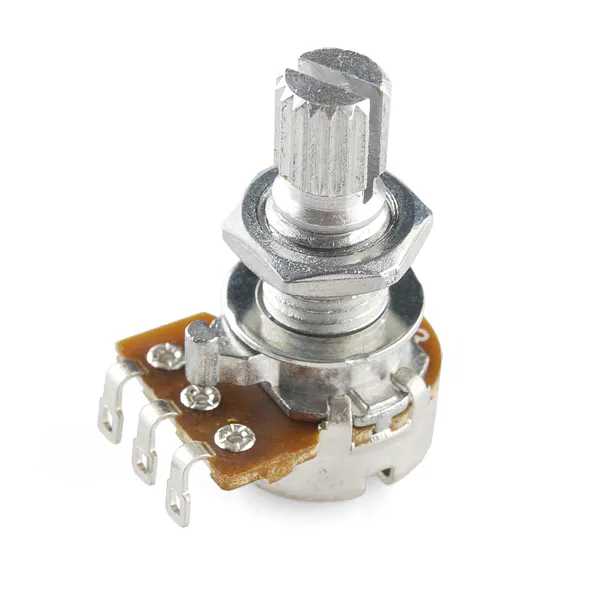Rotary potentiometer (generic)
×1
 Black Metal Knob - 14x24mm
×1
 Piezo Element
×1
 Toggle Switch and Cover - Illuminated (Red)
×3
 Pushbutton 33mm - Green
×2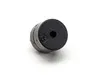Buzzer
×1
 Hobby Motor - Gear
×1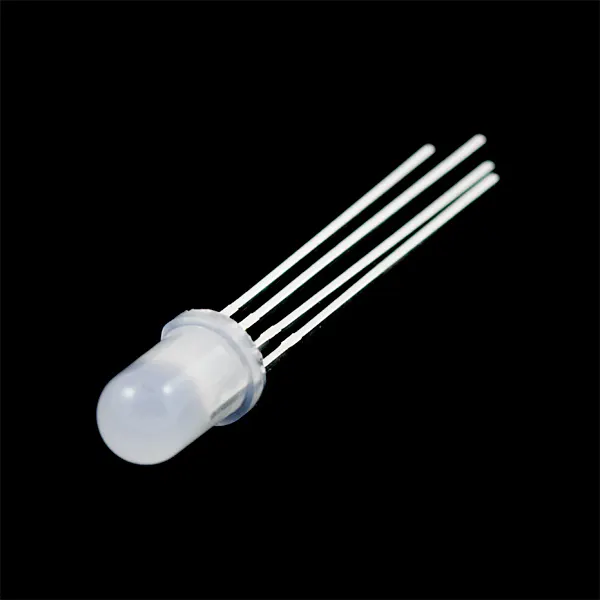RGB Diffused Common Cathode
×6
 7-Segment Display - LED (Red)
×3
 Tupperware Square
×1Arduino UNO & Genuino UNO
×1

### Software apps and online servicesArduino IDE

### Hand tools and fabrication machinesHot glue gun (generic)Soldering iron (generic)
 Wire Stripper
 Drill

## Code

### Interactive_child_toy.ino

C/C++
Contains the code for all the components of the project; Be sure to unplug RX pin before flashing.
```//Interactive Children's Toy - 2017 - Roberto Jacobo and Frank Burke-Olson

//Motor and buzzer pins
int buzzPot = A3;
int buzzPin = 5;
int potValue;

int motorPot = A4;
int motorPin = 6;
int motorValue;
int motortime = 0;

int work = 2; //decides which side is active; 1 = buzzer, 2 = motor, 3 = piezo & led

//Segment display
int a = 0;
int b = 1;
int c = 2;
int d = 3;
int e = 4;
int f = 7;
int g = 8;
int segnum = 0;
int buttplus = 12;
int buttmin = 13;

//Tap and linelight
int knockSensor = A5;
byte val = 0;
int THRESHOLD = 25;
int tapnum = -1;
int redPin = 9;
int greenPin = 10;
int bluePin = 11;
//pins A0-2 correspond to leds 1-3

void setup() {

//led display
pinMode(a, OUTPUT);
pinMode(b, OUTPUT);
pinMode(c, OUTPUT);
pinMode(d, OUTPUT);
pinMode(e, OUTPUT);
pinMode(f, OUTPUT);
pinMode(g, OUTPUT);
pinMode(buttplus, INPUT);
pinMode(buttmin, INPUT);

//tap and linelight
pinMode(A0, OUTPUT);
pinMode(A1, OUTPUT);
pinMode(A2, OUTPUT);
pinMode(knockSensor, INPUT);
pinMode(redPin, OUTPUT);
pinMode(greenPin, OUTPUT);
pinMode(bluePin, OUTPUT);

}

void loop() {
//BUZZER
if(work==1){
if(hasChangedBuzzer(buzzPot))
tone(buzzPin,newVal,1000);
else{
noTone(buzzPin);
work=2;
}
}

//MOTOR
if(work==2){
if(hasChangedMotor(motorPot)){
motortime = 0;
motorValue = map(potValue, 50, 1023, 80, 230);
analogWrite(motorPin,motorValue);
}else{
motortime++;
}
if (motortime > 10){
analogWrite(motorPin,0);
motortime = 10;
work = 3;
}
}

//SEG LEDs
printnum(segnum);

segnum++;
if(segnum == 10){
segnum = 0;
}
delay(150);
segnum--;
if(segnum == -1){
segnum = 9;
}
delay(100);
}

//PIEZO TAP
if(work==3){
work =1;
if (val <= THRESHOLD) {
tapnum++;
if(tapnum == 7){
tapnum = 0;
}

if(tapnum == 0){
setColor(255, 0, 0);  // red
}else if(tapnum == 1){
setColor(0, 255, 0);  // green
}else if(tapnum == 2){
setColor(0, 0, 255);  // blue
}else if(tapnum == 3){
setColor(255, 255, 0);  // yellow
}else if(tapnum == 4){
setColor(80, 0, 80);  // purple
}else {
setColor(0, 255, 255);  // aqua
}

digitalWrite(A0,HIGH);
digitalWrite(A1,HIGH);
digitalWrite(A2,HIGH);
digitalWrite(A3,HIGH);

digitalWrite(A0,LOW);
delay(80);
digitalWrite(A1,LOW);
delay(70);
digitalWrite(A2,LOW);
delay(50);
digitalWrite(A2,HIGH);
delay(50);
digitalWrite(A1,HIGH);
delay(70);
digitalWrite(A0,HIGH);
delay(80);

delay(100);
}
}
}

//Sets colors to RGB LEDs
void setColor(int red, int green, int blue)
{
#ifdef COMMON_ANODE
red = 255 - red;
green = 255 - green;
blue = 255 - blue;
#endif
analogWrite(redPin, red);
analogWrite(greenPin, green);
analogWrite(bluePin, blue);
}

//Writes numbers on display
void printnum(int num){

digitalWrite(a, HIGH);
digitalWrite(b, HIGH);
digitalWrite(c, HIGH);
digitalWrite(d, HIGH);
digitalWrite(e, HIGH);
digitalWrite(f, HIGH);
digitalWrite(g, HIGH);

if(num ==0){
digitalWrite(a, LOW);
digitalWrite(b, LOW);
digitalWrite(c, LOW);
digitalWrite(d, LOW);
digitalWrite(e, LOW);
digitalWrite(f, LOW);

}else if(num ==1){
digitalWrite(b, LOW);
digitalWrite(c, LOW);

}else if(num ==2){
digitalWrite(a, LOW);
digitalWrite(b, LOW);
digitalWrite(g, LOW);
digitalWrite(e, LOW);
digitalWrite(d, LOW);

}else if(num ==3){
digitalWrite(a, LOW);
digitalWrite(b, LOW);
digitalWrite(c, LOW);
digitalWrite(d, LOW);
digitalWrite(g, LOW);

}else if(num ==4){
digitalWrite(b, LOW);
digitalWrite(c, LOW);
digitalWrite(f, LOW);
digitalWrite(g, LOW);

}else if(num ==5){
digitalWrite(a, LOW);
digitalWrite(c, LOW);
digitalWrite(d, LOW);
digitalWrite(f, LOW);
digitalWrite(g, LOW);

}else if(num ==6){
digitalWrite(a, LOW);
digitalWrite(c, LOW);
digitalWrite(d, LOW);
digitalWrite(e, LOW);
digitalWrite(f, LOW);
digitalWrite(g, LOW);

}else if(num ==7){
digitalWrite(a, LOW);
digitalWrite(b, LOW);
digitalWrite(c, LOW);

}else if(num ==8){
digitalWrite(a, LOW);
digitalWrite(b, LOW);
digitalWrite(c, LOW);
digitalWrite(d, LOW);
digitalWrite(e, LOW);
digitalWrite(f, LOW);
digitalWrite(g, LOW);

}else {
digitalWrite(a, LOW);
digitalWrite(b, LOW);
digitalWrite(c, LOW);
digitalWrite(d, LOW);
digitalWrite(f, LOW);
digitalWrite(g, LOW);

}
}

//Tests if buzzer potentiometer has changed
boolean hasChangedBuzzer(int pin) {
delay(20);
int difference = abs(potValNew - potValInit);
if (difference > 17) {
return true;
}
else {
return false;
}
}

//Tests if motor potentiometer has changed
boolean hasChangedMotor(int pin){
delay(100);
int difference = abs(valInit-valNew);
if(difference > 60){
return true;
}
else{
valInit = valNew;
return false;
}
}
```

## Credits

### Frank Burke-Olson

2 projects • 7 followers

### Roberto Jacobo

2 projects • 6 followers
Junior at Lane Tech